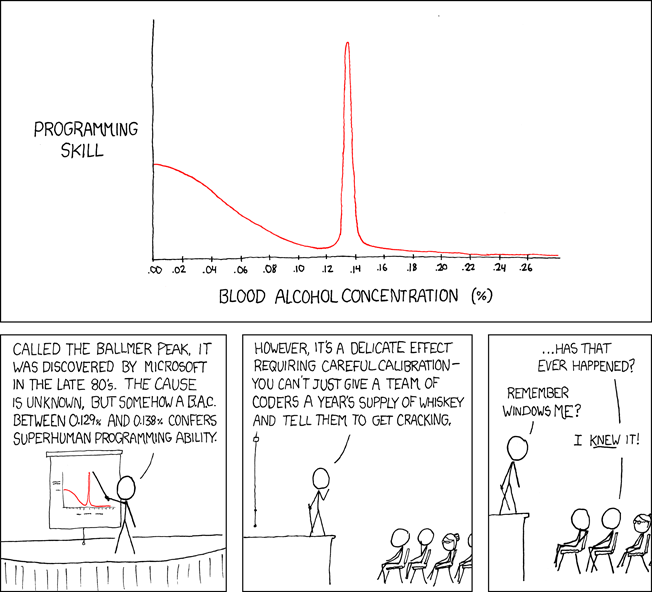2015-01-31
ReactJS and the Ballmer Peak

My previous post showed how ReactJS and AngularJS can coexist in the same page. I used a simple example of each to show a periodically updating timer display. Now I’m going to try to build a more useful and powerful ReactJS example, the Ballmer Peak Calculator.

The XKCD author’s definition of Ballmer Peak has become the defacto illustration of the concept. The image below was obtained from XKCD - Ballmer Peak:#### The ReactJS Ballmer Peak Example

I obtained the React Starter Kit 0.12.2 from the Getting Started page. On this page, I’m going to try to implement the Ballmer Peak example copied from the examples/ballmer-peak/ directory.

I’m adapting it to a more blog-accessible form by inlining the Javascript original example.js into this page. I also moved non-dynamic, non-reactive content out of the example and into the Markdown above. I needed to use <script type="text/jsx"> to invoke the JSX translator, but that works fine except for a Javascript warning suggesting I precompile my JSX.

The code for the above is:

<h4>Ballmer Peak Calculator in ReactJS</h4>
<div id="container" class="card bg-well p-1">
<p>
ReactJS is not working if you see this text.
</p>
</div>

<script type="text/jsx">
function computeBallmerPeak(x) {
x = x * 100;
return (
1-1/(1+Math.exp(-(x-6)))*.5 + Math.exp(-Math.pow(Math.abs(x-10), 2)*10)
) / 1.6;
}

function percentage(x) {
return isNaN(x) ? 'N/A' : (100 - Math.round(x * 100)) + '%';
}

var BallmerPeakCalculator = createReactClass({
getInitialState: function() {
return {bac: 0};
},
handleChange: function(event) {
this.setState({bac: event.target.value});
},
render: function() {
var pct = percentage(computeBallmerPeak(this.state.bac));
return (
<div>
<p>
<input type="text" onChange={this.handleChange} value={this.state.bac} />
{', '}then <b>{pct}</b> of your lines of code will be bug free.
</p>
</div>
);
}
});

ReactDOM.render(
<BallmerPeakCalculator />,
document.getElementById('container')
);


#### Ballmer Peak in AngularJS

Let’s write the equivalent of the above in Angular. Note that we don’t even need an explicit controller here; AngularJS is sufficient for this example but we’d have to inline a bunch of math into the HTML. So I’m going to use a simple inline controller and place the percentage and computeBallmerPeak functions there.

The code for the above is:

<h4>Ballmer Peak Calculator in AngularJS</h4>
<div id="container" class="card bg-well p-1" ng-controller="ControllerA">
<div>
<p>
<input type="text" ng-model="BAC"></input>
then <b>{{ percentage(computeBallmerPeak(BAC)) }}</b> of your lines of code will be bug free.
</p>
</div>
</div>

<script>
function ControllerA($scope) {$scope.BAC = 0;
$scope.computeBallmerPeak = function(x) { // see: http://ask.metafilter.com/76859/Make-a-function-of-this-graph-Thats-like-an-antigraph x = x * 100; return ( 1-1/(1+Math.exp(-(x-6)))*.5 + Math.exp(-Math.pow(Math.abs(x-10), 2)*10) ) / 1.6; };$scope.percentage = function(x) {
return isNaN(x) ? 'N/A' : (100 - Math.round(x * 100)) + '%';
};
}
angular
.module('BlogApp')
.controller('ControllerA', ControllerA);
</script>